# Minimum path sum in Triangular Grid (DP 11)

In this article, we will solve the most asked coding interview problem: Minimum path sum in Triangular Grid

Problem Link: Fixed Starting and Ending Point

Problem Description:

We are given a Triangular matrix. We need to find the minimum path sum from the first row to the last row.

At every cell we can move in only two directions: either to the bottom cell (↓) or to the bottom-right cell(↘)

```Example: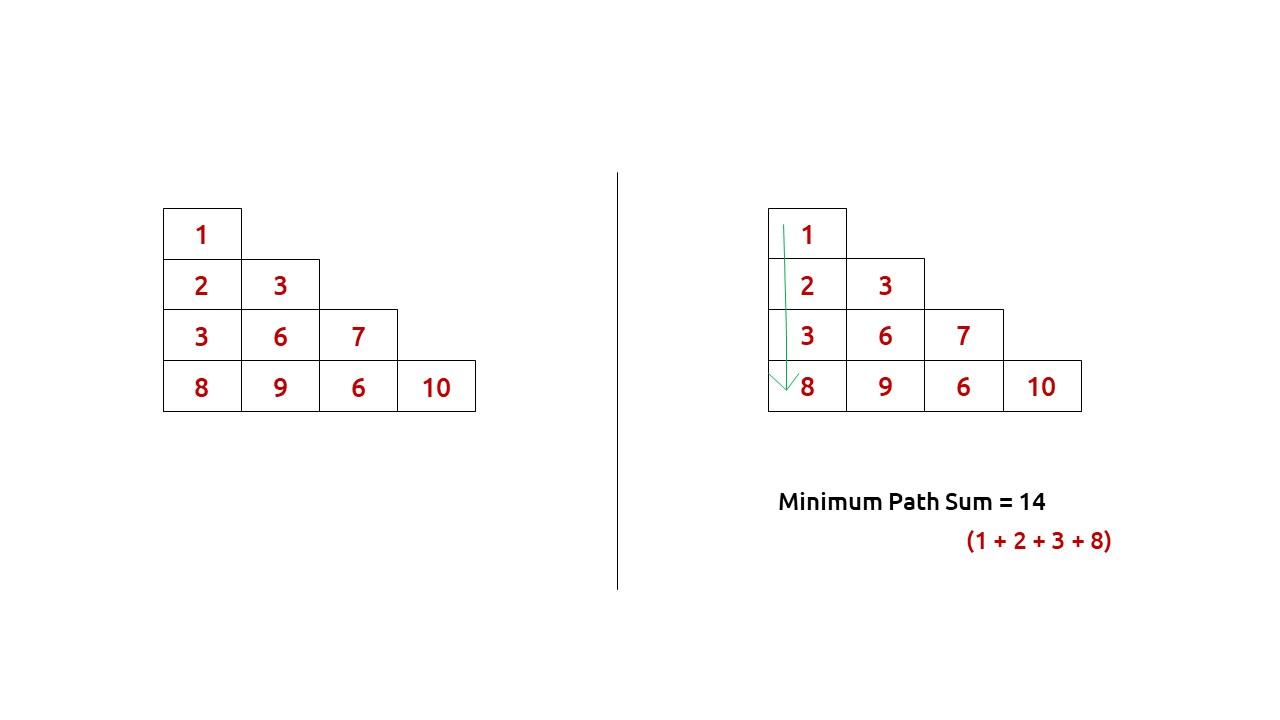```

Disclaimer: Don’t jump directly to the solution, try it out yourself first.

Pre-req: Minimum Path Sum in a Grid

### Solution :

This question is a slight modification of the question discussed in Minimum Path Sum in a Grid. In the previous problem, we were given a rectangular matrix whereas here the matrix is in the form of a triangle. Moreover, we don’t have a fixed destination, we need to return the minimum sum path from the top cell to any cell of the bottom row.

## Why a Greedy Solution doesn’t work?

As we have to return the minimum path sum, the first approach that comes to our mind is to take a greedy approach and always form a path by locally choosing the cheaper option.

This approach will not give us the correct answer. Let us look at this example to understand: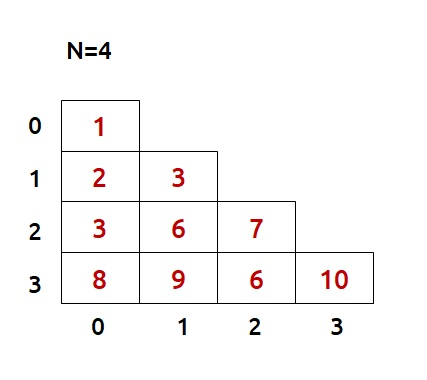At every cell, we have two choices: to move to the bottom cell or move to the bottom-right cell. Our ultimate aim is to provide a path that provides us the least path sum. Therefore at every cell, we will make the choice to move which costs us less.

• Figure on the left gives us a greedy solution, where we move by taking the local best choice.
• Figure on the right gives us a non-greedy solution.

We can clearly see the problem with the greedy solution. Whenever we are making a local choice, we may tend to choose a path that may cost us way more later.

Therefore, the other alternative left to us is to generate all the possible paths and see which is the path with the minimum path sum. To generate all paths we will use recursion.

## Steps to form the recursive solution:

We will first form the recursive solution by the three points mentioned in Dynamic Programming Introduction

Step 1: Express the problem in terms of indexes.

We are given a triangular matrix with the number of rows equal to N.

We can define the function with two parameters i and j, where i and j represent the row and column of the matrix.

Now our ultimate aim is to reach the last row. We can define f(i,j) such that it gives us the minimum path sum from the cell [i][j] to the last row.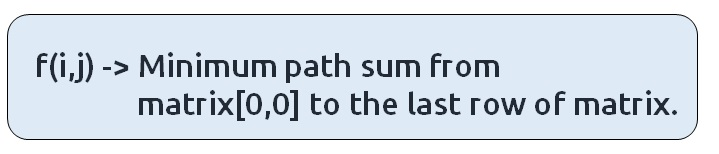We want to find f(0,0) and return it as our answer.

Base Case:

There will be a single base case:

• When i == N-1, that is when we have reached the last row, so the min path from that cell to the last row will be the value of that cell itself, hence we return mat[i][j].

At every cell, we have two options to move to the bottom cell(↓) or to the bottom-right cell(↘). If we closely observe the triangular grid, at max we can reach the last row from where we return so we will not be able to go move out of the index of the grid. Therefore only one base condition is required.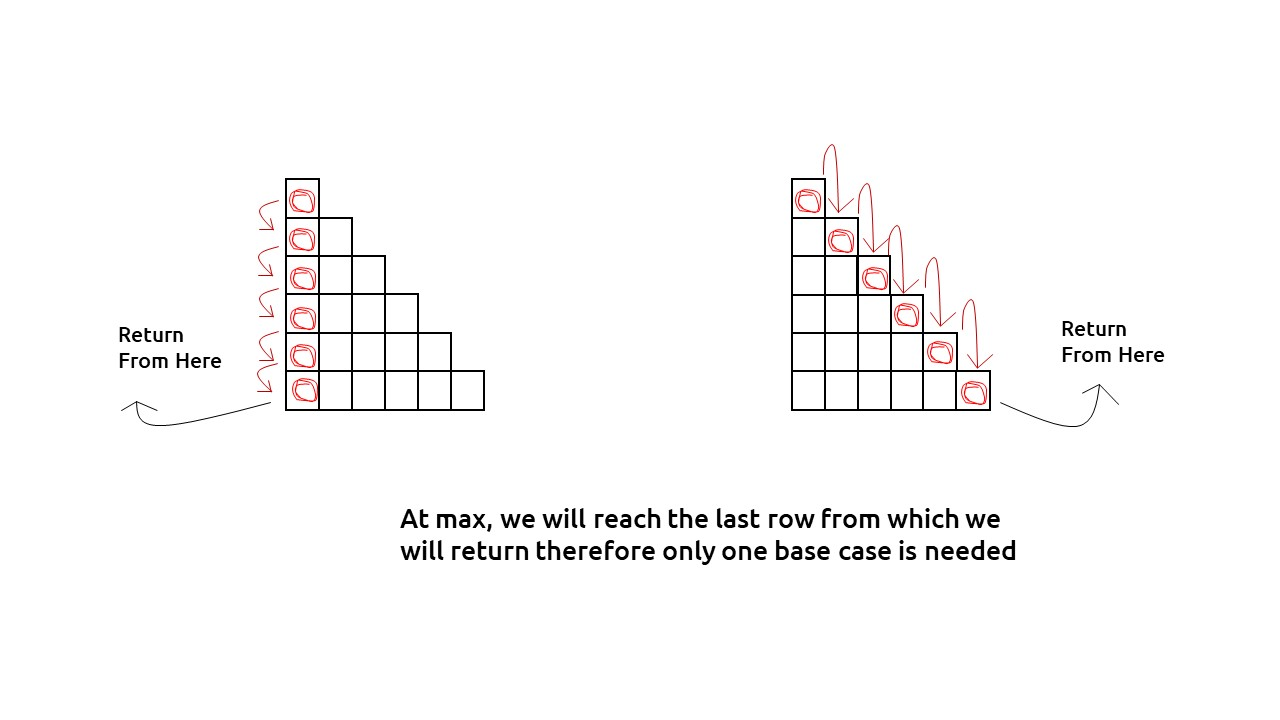The pseudocode till this step will be: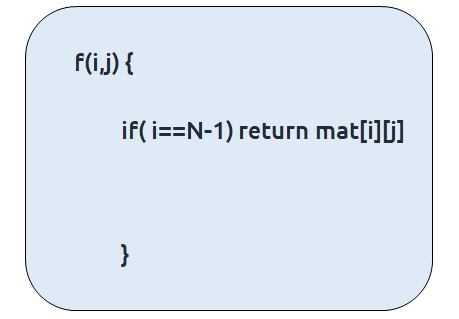Step 2: Try out all possible choices at a given index.

At every index we have two choices, one to go to the bottom cell(↓) other to the bottom-right cell(↘). To go to the bottom, we will increase i by 1, and to move towards the bottom-right we will increase both i and j by 1.

Now when we get our answer for the recursive call (f(i+1,j) or f(i+1,j+1)), we need to also add the current cell value to it as we have to include it too for the current path sum.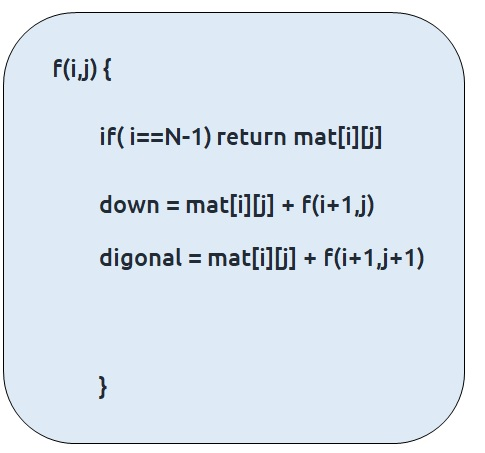Step 3:  Take the maximum of all choices

As we have to find the minimum path sum of all the possible unique paths, we will return the minimum of the choices(down and diagonal)

The final pseudocode after steps 1, 2, and 3: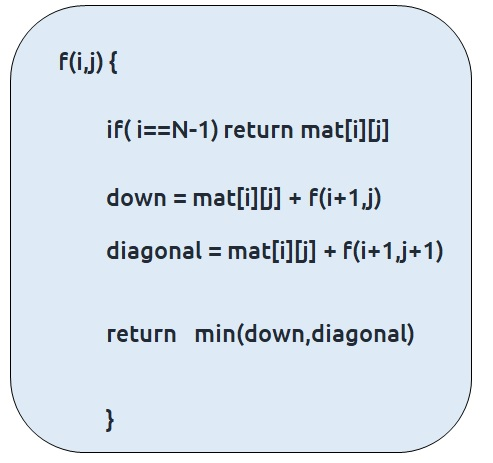## Steps to memoize a recursive solution:

If we draw the recursion tree, we will see that there are overlapping subproblems. In order to convert a recursive solution the following steps will be taken:

1. Create a dp array of size [N][N]
2. Whenever we want to find the answer of a particular row and column (say f(i,j)), we first check whether the answer is already calculated using the dp array(i.e dp[i][j]!= -1 ). If yes, simply return the value from the dp array.
3. If not, then we are finding the answer for the given values for the first time, we will use the recursive relation as usual but before returning from the function, we will set dp[i][j] to the solution we get.

Code:

## C++ Code

``````#include <bits/stdc++.h>

using namespace std;

int minimumPathSumUtil(int i, int j, vector<vector<int> > &triangle,int n,
vector<vector<int> > &dp) {

if(dp[i][j]!=-1)
return dp[i][j];

if(i==n-1) return triangle[i][j];

int down = triangle[i][j]+minimumPathSumUtil(i+1,j,triangle,n,dp);
int diagonal = triangle[i][j]+minimumPathSumUtil(i+1,j+1,triangle,n,dp);

return dp[i][j] = min(down, diagonal);

}

int minimumPathSum(vector<vector<int> > &triangle, int n){
vector<vector<int> > dp(n,vector<int>(n,-1));
return minimumPathSumUtil(0,0,triangle,n,dp);

}

int main() {

vector<vector<int> > triangle{{1},
{2,3},
{3,6,7},
{8,9,6,10}};

int n = triangle.size();

cout<<minimumPathSum(triangle,n);
}
``````

Output: 14

Time Complexity: O(N*N)

Reason: At max, there will be (half of, due to triangle) N*N calls of recursion.

Space Complexity: O(N) + O(N*N)

Reason: We are using a recursion stack space: O((N), where N is the path length and an external DP Array of size ‘N*N’.

## Java Code

``````import java.util.*;
class TUF{
static int minimumPathSumUtil(int i, int j, int[][] triangle,int n,int[][] dp) {

if(dp[i][j]!=-1)
return dp[i][j];

if(i==n-1) return triangle[i][j];

int down = triangle[i][j]+minimumPathSumUtil(i+1,j,triangle,n,dp);
int diagonal = triangle[i][j]+minimumPathSumUtil(i+1,j+1,triangle,n,dp);

return dp[i][j] = Math.min(down, diagonal);

}

static int minimumPathSum(int[][] triangle, int n){
int dp[][]=new int[n][n];
for(int row[]: dp)
Arrays.fill(row,-1);
return minimumPathSumUtil(0,0,triangle,n,dp);

}

public static void main(String args[]) {

int triangle [][] = {{1},
{2,3},
{3,6,7},
{8,9,6,10}};

int n = triangle.length;

System.out.println(minimumPathSum(triangle,n));
}
}
``````

Output: 14

Time Complexity: O(N*N)

Reason: At max, there will be (half of, due to triangle) N*N calls of recursion.

Space Complexity: O(N) + O(N*N)

Reason: We are using a recursion stack space: O((N), where N is the path length and an external DP Array of size ‘N*N’.

## Steps to convert Recursive Solution to Tabulation one.

Recursion/Memoization is a top-down approach whereas tabulation is a bottom-up approach. As in recursion/memoization, we have moved from 0 to N-1, in tabulation we move from N-1 to 0, i.e last row to the first one.

The steps to convert to the tabular solution are given below:

• Declare a dp[] array of size [N][N].
• First initialize the base condition values, i.e the last row of dp matrix to the last row of the triangle matrix.
• Our answer should get stored in dp. We want to move from the last row to the first row. So that whenever we compute values for a cell, we have all the values required to calculate it.
• If we see the memoized code, values required for dp[i][j] are: dp[i+1][j] and dp[i+1][j+1]. So we only need the values from the ‘i+1’ row.
• We have already filled the last row (i=N-1), if we start from row ‘N-2’ and move upwards we will find the values correctly.
• We can use two nested loops to have this traversal.

Code:

## C++ Code

``````#include <bits/stdc++.h>

using namespace std;

int minimumPathSum(vector<vector<int> > &triangle, int n){
vector<vector<int> > dp(n,vector<int>(n,0));

for(int j=0;j<n;j++){
dp[n-1][j] = triangle[n-1][j];
}

for(int i=n-2; i>=0; i--){
for(int j=i; j>=0; j--){

int down = triangle[i][j]+dp[i+1][j];
int diagonal = triangle[i][j]+dp[i+1][j+1];

dp[i][j] = min(down, diagonal);
}
}

return dp;

}

int main() {

vector<vector<int> > triangle{{1},
{2,3},
{3,6,7},
{8,9,6,10}};

int n = triangle.size();

cout<<minimumPathSum(triangle,n);
}
``````

Output:

21

Time Complexity: O(N*N)

Reason: There are two nested loops

Space Complexity: O(N*N)

Reason: We are using an external array of size ‘N*N’. The stack space will be eliminated.

## Java Code

``````import java.util.*;
class TUF{
static int minimumPathSum(int[][] triangle, int n){
int dp[][]= new int[n][n];

for(int j=0;j<n;j++){
dp[n-1][j] = triangle[n-1][j];
}

for(int i=n-2; i>=0; i--){
for(int j=i; j>=0; j--){

int down = triangle[i][j]+dp[i+1][j];
int diagonal = triangle[i][j]+dp[i+1][j+1];

dp[i][j] = Math.min(down, diagonal);
}
}

return dp;

}

public static void main(String args[]) {

int triangle [][] = {{1},
{2,3},
{3,6,7},
{8,9,6,10}};

int n = triangle.length;

System.out.println(minimumPathSum(triangle,n));
}
}
``````

Output:

21

Time Complexity: O(N*N)

Reason: There are two nested loops

Space Complexity: O(N*N)

Reason: We are using an external array of size ‘N*N’. The stack space will be eliminated.

## Part 3: Space Optimization

If we closely look the relation,

dp[i][j] = matrix[i][j] + min(dp[i+1][j] + dp[i+1][j+1]))

We see that we only need the next row, in order to calculate dp[i][j]. Therefore we can space optimize it.

Initially we can take a dummy row ( say front). We initialize this row to the triangle matrix last row( as done in tabulation).

Now the current row(say cur) only needs the front row’s value in order to calculate dp[i][j].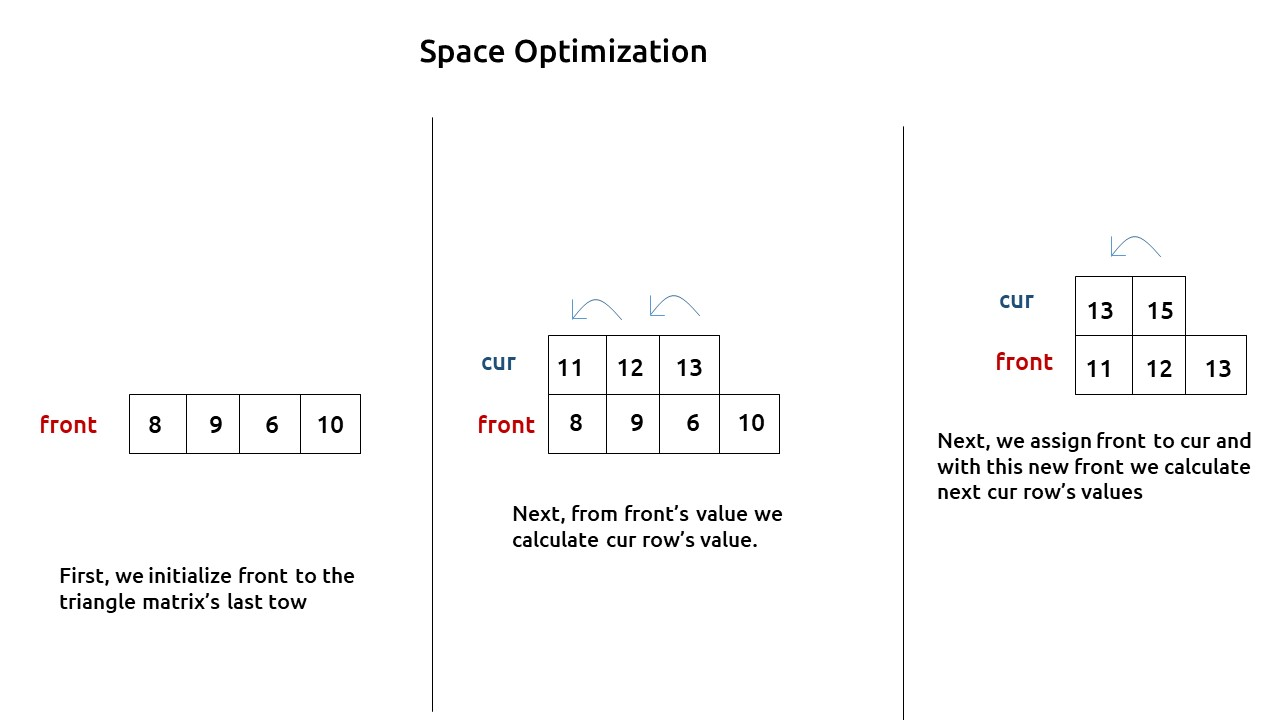At last, front  will give us the required answer.

Code:

## C++ Code

``````#include <bits/stdc++.h>

using namespace std;

int minimumPathSum(vector<vector<int> > &triangle, int n){
vector<int> front(n,0), cur(n,0);

for(int j=0;j<n;j++){
front[j] = triangle[n-1][j];
}

for(int i=n-2; i>=0; i--){
for(int j=i; j>=0; j--){

int down = triangle[i][j]+front[j];
int diagonal = triangle[i][j]+front[j+1];

cur[j] = min(down, diagonal);
}
front=cur;
}

return front;

}

int main() {

vector<vector<int> > triangle{{1},
{2,3},
{3,6,7},
{8,9,6,10}};

int n = triangle.size();

cout<<minimumPathSum(triangle,n);
}
``````

Output:

14

Time Complexity: O(N*N)

Reason: There are two nested loops

Space Complexity: O(N)

Reason: We are using an external array of size ‘N’ to store only one row.

## Java Code

``````import java.util.*;
class TUF{
static int minimumPathSum(int[][] triangle, int n){

int front[] = new int[n];
int cur[] = new int[n];

for(int j=0;j<n;j++){
front[j] = triangle[n-1][j];
}

for(int i=n-2; i>=0; i--){
for(int j=i; j>=0; j--){

int down = triangle[i][j]+front[j];
int diagonal = triangle[i][j]+front[j+1];

cur[j] = Math.min(down, diagonal);
}
front=cur;
}

return front;

}

public static void main(String args[]) {

int triangle [][] = {{1},
{2,3},
{3,6,7},
{8,9,6,10}};

int n = triangle.length;

System.out.println(minimumPathSum(triangle,n));
}
}
``````

Output:

14

Time Complexity: O(N*N)

Reason: There are two nested loops

Space Complexity: O(N)

Reason: We are using an external array of size ‘N’ to store only one row.

Special thanks to Anshuman Sharma for contributing to this article on takeUforward. If you also wish to share your knowledge with the takeUforward fam, please check out this article